# Сборник текстов на казахском, русском, английском языках для формирования навыков по видам речевой деятельности обучающихся уровней среднего образования

 бет 23/65 Дата 05.11.2016 өлшемі 17,36 Mb.

## Ionic Bonds

Ionic bonds form when two atoms have a large difference in electronegativity. (Electronegativity is the quantitative representation of an atom’s ability to attract an electron to itself). Although scientists do not have an exact value to signal an ionic bond, the amount is generally accepted as 1.7 and over to qualify a bond as ionic. Ionic bonds often occur between metals and salts; chloride is often the bonding salt. Compounds displaying ionic bonds form ionic crystals in which ions of positive and negative charges hover near each other, but there is not always a direct 1-1 correlation between positive and negative ions. Ionic bonds can typically be broken through hydrogenation, or the addition of water to a compound.

## Covalent Bonds

Covalent bonds form when two atoms have a very small (nearly insignificant) difference in electronegativity. The value of difference in electronegativity between two atoms in a covalent bond is less than 1.7. Covalent bonds often form between similar atoms, nonmetal to nonmetal or metal to metal. Covalent bonding signals a complete sharing of electrons. There is usually a direct correlation between positive and negative ions, meaning that because they share electrons, the atoms balance. Covalent bonds are usually strong because of this direct bonding.

## Polar Covalent Bonds

Polar covalent bonds fall between ionic and covalent bonds. They result when two elements bond with a moderate difference in electronegativity moderately to greatly, but they do not surpass 1.7 in electronegativity difference. Although polar covalent bonds are classified as covalent, they do have significant ionic properties. They also induce dipole-dipole interactions, where one atom becomes slightly negative and the other atom becomes slightly positive. However, the slight change in charge is not large enough to classify it entirely as an ion; they are simply considered slightly positive or slightly negative. Polar covalent bonds often indicate polar molecules, which are likely to bond with other polar molecules but are unlikely to bond with non-polar molecules.

## Hydrogen Bonds

Hydrogen bonds only form between hydrogen and oxygen (O), nitrogen (N) or fluorine (F). Hydrogen bonds are very specific and lead to certain molecules having special properties due to these types of bonds. Hydrogen bonding sometimes results in the element that is not hydrogen (oxygen, for example) having a lone pair of electrons on the atom, making it polar. Lone pairs of electrons are non-bonding electrons that sit in twos (pairs) on the central atom of the compound. Water, for example, exhibits hydrogen bonding and polarity as a result of the bonding. This is shown in the diagram below.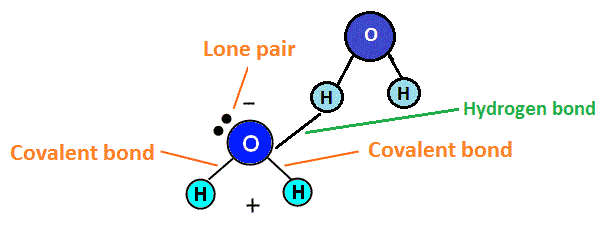Because of this polarity, the oxygen end of the molecule would repel negative atoms like itself, while attracting positive atoms, like hydrogen. Hydrogen, which becomes slightly positive, would repel positive atoms (like other hydrogen atoms) and attract negative atoms (such as oxygen atoms). This positive and negative attraction system helps water molecules stick together, which is what makes the boiling point of water high (as it takes more energy to break these bonds between water molecules).

In addition to the four types of chemical bonds, there are also three categories bonds fit into: single, double, and triple. Single bonds involve one pair of shared electrons between two atoms. Double bonds involve two pairs of shared electrons between two atoms, and triple bonds involve three pairs of shared electrons between two atoms. These bonds take on different natures due to the differing amounts of electrons needed and able to be given up.

Now, let’s look at determining what types of bonds we see in different compounds. We’ve already looked at the bonds in H2O, which we determined to be hydrogen bonds. However, now let’s look at a few other types of bonds as examples.

Compound: HNO3 (also known as Nitric acid)

There are two different determinations we can make as to what these bonds look like; first we can decide whether the bonds are covalent, polar covalent, ionic, or hydrogen. Then, we can determine if the bonds are single, double, or triple.

In order to decide whether the bonds are covalent, polar covalent, ionic or hydrogen, we need to look at the types of elements seen and the electronegativity values. We look at the elements and see hydrogen, nitrogen, and oxygen—no metals. This rules out ionic bonding as a type of bond seen in the compound. Then, we would look at electronegativity values for nitrogen and oxygen. Oftentimes, this information can be found on a periodic table, in a book index, or an educational online resource. The electronegativity value for oxygen is 3.5 and the electronegativity value for nitrogen is 3.0. The way to determine the bond type is by taking the difference between the two numbers (subtraction). 3.5 – 3.0 = 0.5, so we can determine that the bond between nitrogen and oxygen is a covalent bond. We can also determine, from past knowledge, that the bond between oxygen and hydrogen is a hydrogen bond as it was in water.

Now, we need to count the electrons and draw the diagram for HNO3. For more help counting electrons, please see the page on Electron Configuration. For more help drawing the Lewis structures, please see the page on Lewis Structures. This process combines both of these in order to determine the structure and shape of a molecule of the compound.

First, we determine that N follows the octet rule, so it needs eight surrounding electrons. This is important to keep in mind as we move forward. Next we count up how many valence electrons the compound has as a whole. H gives us 1, N gives us 5, and each O gives us 6. We can discern this from looking at the tops of the columns in the periodic table (see above). We then add these numbers together (3 x 6 = 18, + 1 = 19, + 5 = 24), and we get 24 electrons that we need to distribute throughout the molecule. First, we need to draw the molecule to see how many initial bonds we’ll be putting in. Our preliminary structure looks like this: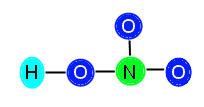Now, we can count how many electrons we have used by counting 2 electrons for each bond placed. We see that we have placed 4 bonds, so we have used 8 electrons. 24 – 8 = 16 electrons that we need to distribute. In order to correctly place the rest of the electrons, we need to determine how many electrons each atom needs to be stable.

The central atom, N, has three bonds attached (equivalent of 6 electrons) so it needs 2 more electrons to be stable. The O to the right has one bond (two electrons) so it needs 6 more to be stable. The O above the N has one bond (two electrons) so it also needs 6 electrons to be stable. The O to the left of the N is bonded both to N and to H, so it has two bonds (4 electrons); therefore, it needs 4 more electrons to be stable. We add up the total amount of electrons needed, 2 + 6 + 6 + 4 = 18, and see that we need 18 electrons to stabilize the compound. We know this is not possible, since we only have 16 available electrons. When this happens, we need to insert a double bond in order to resolve the problem of lack of electrons. This is because, although we count each bond as 2 electrons, the elements joined together in the bond are actually sharing the electrons. Therefore, when we count out the bonds, we are counting some electrons twice because they are shared. This is normal and expected, and resolves not having enough valence electrons. Now, we need to decide where to put the double bond in this compound. We know that the double bond cannot go between O and H, because H does not have enough room to accept another electron. Therefore, we know we must place the bond between N and O. You might be thinking, how do I decide where to put the bond? In this particular example, we can place the bond either between the top O and N, or the right O and N. This is because HNO3 displays resonance.

Here are the ways you can place the double bond: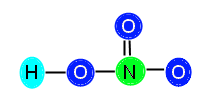or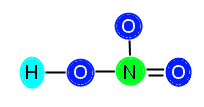We are going to keep the bond between N and the right O in our example. After we add in the bond, we subtract two more electrons from our available electrons (16) and are left with 14 electrons to distribute. Now we need to make sure we have the correct number of electrons. After placing in the double bond, N is now stable because it has 4 bonds (8 electrons) surrounding it. It does not need any additional electrons. The top O (above N) needs 6 electrons, the right O now only needs 4 electrons (because it has a double bond now, which is 4 electrons), and the left O still needs 4 electrons to become stable. We add these numbers together, 6 + 4 + 4 = 14, and we see that 14 is the number of electrons we have, so we can go ahead and distribute them, like this: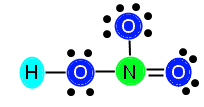Now, our compound is stable with appropriately distributed valence electrons. We can see that there are three single bonds (H—O, N—O, and N—O) and one double bond (N==O). 

Periodic Law and Periodic System

The early innovation of Periodic law by a Russian chemist, Dmitry I. Mendeleev in the mid-19th century, has been of great value in the growth of chemistry. In chemistryPeriodic law is the arrangement of theelements in order of increasing atomic number, that is, the total number of protons in the atomic nucleus and their physical and chemical properties recur in a pattern with the increasing atomic number. In the periodic table, the horizontal rows, known as the “periods” exemplify these relations. In fact, until the second decade of the 20th century, it was not documented that the order ofelements in the periodic system is that of their atomic numbers.

Достарыңызбен бөлісу: